• +91 9971497814
• info@interviewmaterial.com

# RD Chapter 10- Congruent Triangles Ex-10.3 Interview Questions Answers

### Related Subjects

Question 1 : In the figure, lines l1 and l2 intersect at O, forming angles as shown in the figure. If x = 45, find the values of y, z and n.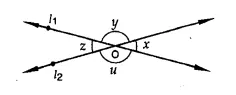Two lines l1 and l2 intersect each other at O ∠x = 45°
∵ ∠z = ∠x (Vertically opposite angles)
= 45°
But x + y = 180° (Linear pair)
⇒45° + y= 180°
⇒ y= 180°-45°= 135°
But u = y (Vertically opposite angles)
∴ u = 135°
Hence y = 135°, z = 45° and u = 135°

Question 2 : In the figure, three coplanar lines intersect at a point O, forming angles as shown in the figure. Find the values of x, y, z and u.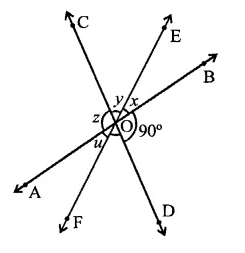Three lines AB, CD and EF intersect at O
∠BOD = 90°, ∠DOF = 50°
∵ AB is a line
∴ ∠BOD + ∠DOF + FOA = 180°
⇒ 90° + 50° + u = 180°
⇒ 140° + w = 180°
∴ u= 180°- 140° = 40°
But x = u (Vertically opposite angles)
∴ x = 40°
Similarly, y = 50° and z = 90°
Hence x = 40°, y = 50°, z = 90° and u = 40°

Question 3 : In the figure, find the values of x, y and z.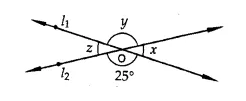Two lines l1 andl2 intersect eachother at O
Vertically opposite angles are equal,
y =25° and x = z
Now 25° + x = 180° (Linear pair)
x=180°-25°= 155°
z = x= 155°
Hence x = 155°, y = 25°, z = 155°

Question 4 : In the figure, find the value of x.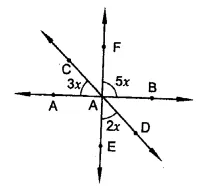∵ EF and CD intersect each other at O
∴ Vertically opposite angles are equal,
∴ ∠1 = 2x
AB is a line
3x + ∠1 + 5x = 180° (Angles on the same side of a line)
⇒ 3x + 2x + 5x = 180°
⇒ 10x = 180° ⇒ x =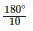= 18°
Hence x = 18°

Question 5 : If one of the four angles formed by two intersecting lines is a right angle, then show that each of the four angles is a right angle.

Given : Two lines AB and CD intersect each other at O. ∠AOC = 90°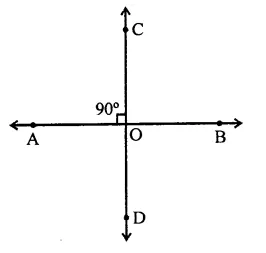To prove: ∠AOD = ∠BOC = ∠BOD = 90°
Proof : ∵ AB and CD intersect each other at O
∴ ∠AOC = ∠BOD and ∠BOC = ∠AOD (Vertically opposite angles)
∴ But ∠AOC = 90°
∴ ∠BOD = 90°
∴ ∠AOC + ∠BOC = 180° (Linear pair)
⇒ 90° + ∠BOC = 180°
∴ ∠BOC = 180° -90° = 90°
∴ ∠AOD = ∠BOC = 90°
∴ ∠AOD = ∠BOC = ∠BOD = 90°

Question 6 :
In the figure, rays AB and CD intersect at O.
(i) Determine y when x = 60°
(ii) Determine x when y = 40°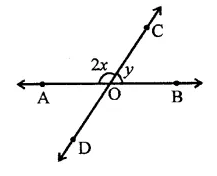In the figure,
AB is a line
∴ 2x + y = 180° (Linear pair)
(i) If x = 60°, then
2 x 60° + y = 180°
⇒ 120° +y= 180°
∴ y= 180°- 120° = 60°
(ii) If y = 40°, then
2x + 40° = 180°
⇒ 2x = 180° – 40° = 140°
⇒ x=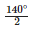=70°
∴ x = 70°

Question 7 : In the figure, lines AB, CD and EF intersect at O. Find the measures of ∠AOC, ∠COF, ∠DOE and ∠BOF.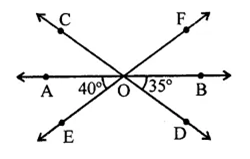Three lines AB, CD and EF intersect each other at O
∠AOE = 40° and ∠BOD = 35°
(i) ∠AOC = ∠BOD (Vertically opposite angles)
= 35°
AB is a line
∴ ∠AOE + ∠DOE + ∠BOD = 180°
⇒ 40° + ∠DOE + 35° = 180°
⇒ 75° + ∠DOE = 180°
⇒ ∠DOE = 180°-75° = 105°
But ∠COF = ∠DOE (Vertically opposite angles)
∴ ∠COF = 105°
Similarly, ∠BOF = ∠AOE (Vertically opposite angles)
⇒ ∠BOF = 40°
Hence ∠AOC = 35°, ∠COF = 105°, ∠DOE = 105° and ∠BOF = 40°

Question 8 : AB, CD and EF are three concurrent lines passing through the point O such that OF bisects ∠BOD. If ∠BOF = 35°, find ∠BOC and ∠AOD.

AB, CD and EF intersect at O. Such that OF is the bisector of
∠BOD ∠BOF = 35°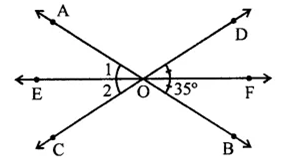∵ OF bisects ∠BOD,
∴ ∠DOF = ∠BOF = 35° (Vertically opposite angles)
∴ ∠BOD = 35° + 35° = 70°
But ∠BOC + ∠BOD = 180° (Linear pair)
⇒ ∠BOC + 70° = 180°
⇒ ∠BOC = 180°-70°= 110°
But ∠AOD = ∠BOC (Vertically opposite angles)
= 110°
Hence ∠BOC = 110° and ∠AOD =110°

Question 9 : In the figure, lines AB and CD intersect at O. If ∠AOC + ∠BOE = 70° and ∠BOD = 40°, find ∠BOE and reflex ∠COE.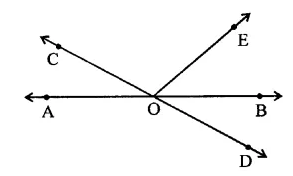In the figure, AB and CD intersect each other at O
∠AOC + ∠BOE = 70°
∠BOD = 40°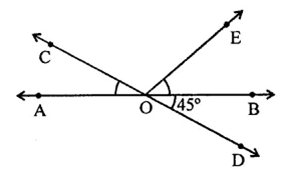AB is a line
∴ ∠AOC + ∠BOE + ∠COE = 180° (Angles on one side of a line)
⇒ 70° + ∠COE = 180°
⇒ ∠COE = 180°-70°= 110°
and ∠AOC = ∠BOD (Vertically opposite angles)
⇒ ∠AOC = 40°
∴ ∠BOE = 70° – 40° = 30°
and reflex ∠COE = 360° – ∠COE
= 360°- 110° = 250°

Question 10 :
Which of the following statements are true (T) and which are false (F)?
(i) Angles forming a linear pair are supplementary.
(ii) If two adjacent angles are equal, then each angle measures 90°.
(iii) Angles forming a linear pair can both be acute angles.
(iv) If angles forming a linear pair are equal, then each of these angles is of measure 90°.

(i) True.
(ii) False. It can be possible if they are a linear pair.
(iii) False. In a linear pair, if one is acute, then the other will be obtuse.
(iv) True.

Todays Deals### RD Chapter 10- Congruent Triangles Ex-10.3 Contributorskrishan

Name:
Email:

# Latest News# 9000 interview questions in different categories